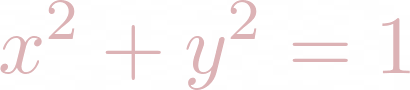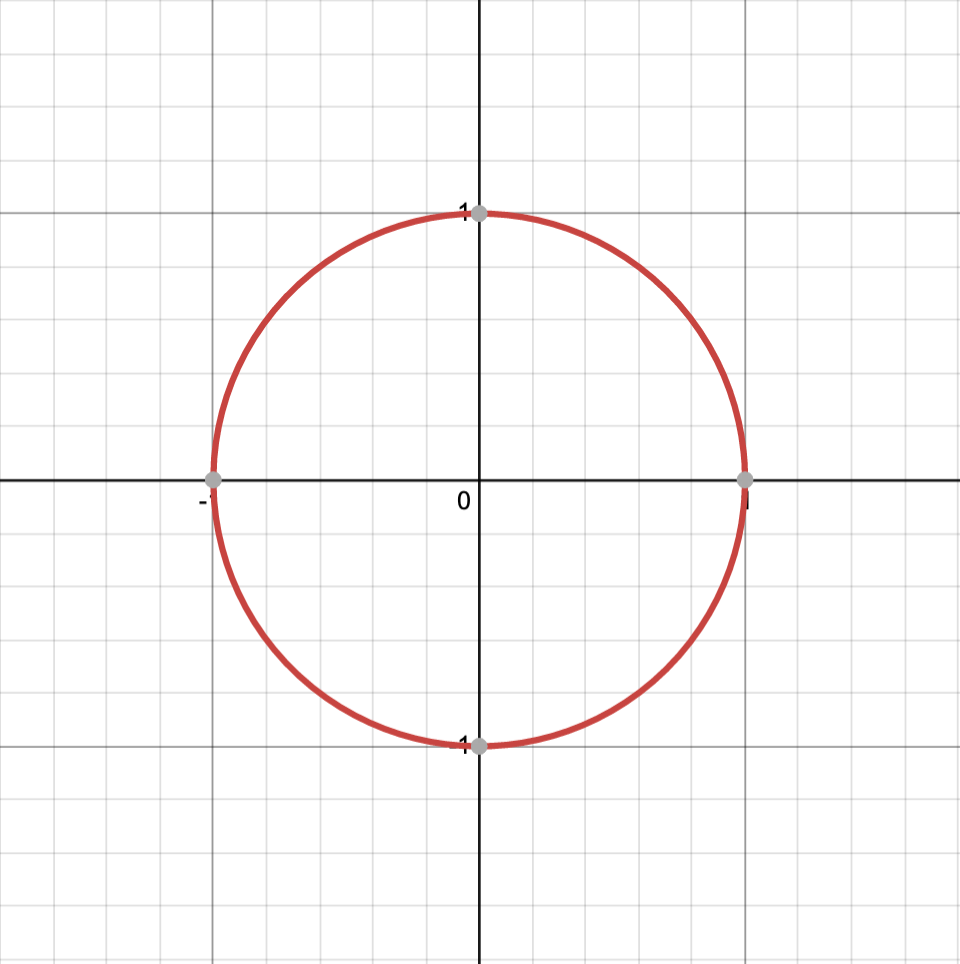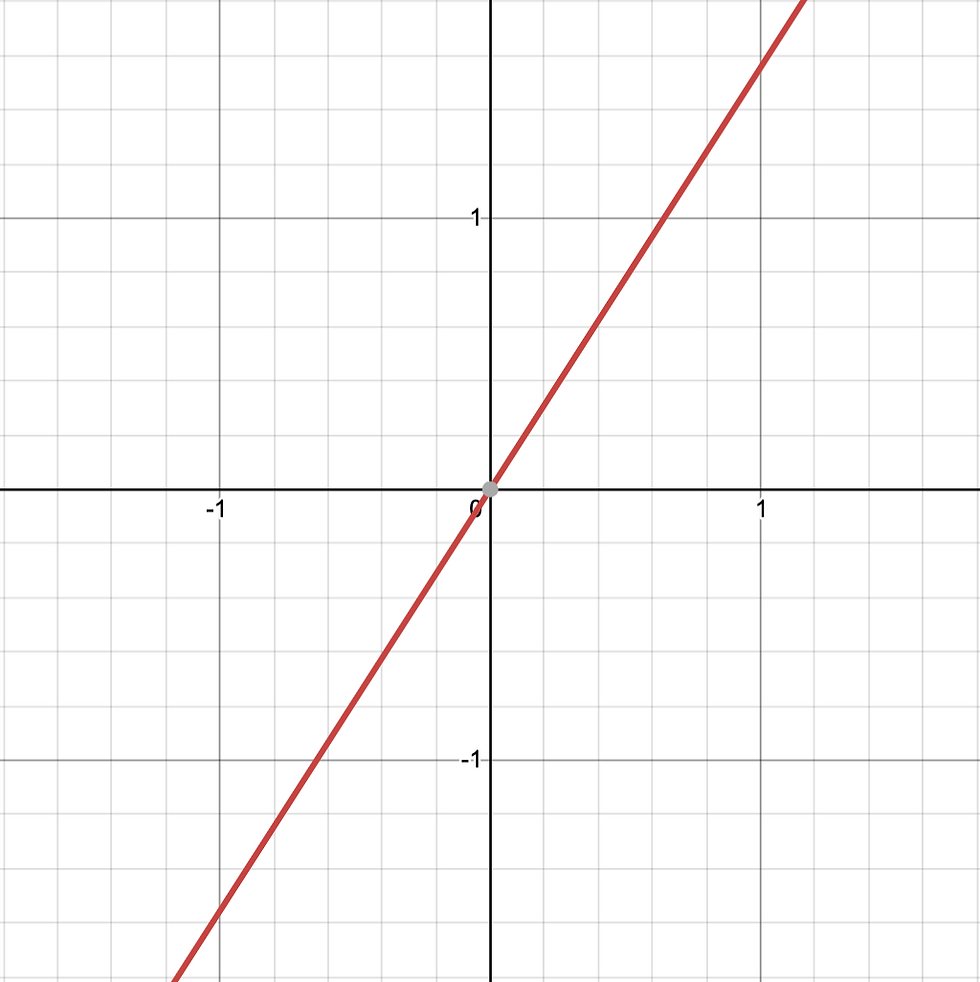top of page# Rectangles in Polar Coordinates

## Circles in rectangular coordinates...Rectangles in polar coordinates?

This is a bit of a goofy one. Why? Well, I want to figure out something very simple using something much more complicated. There are many examples of this sort of thing - and I just find it to be a great aid in understanding the more complicated idea. So let's figure out the area of a square using double integrals in polar coordinates!

The first time we ever learn the equation of a circle, it's in Cartesian coordinates - aka rectangular coordinates. We have the xy-plane and define the unit circle as the set of all points distance 1 from the origin. Using the distance formula, that means we want all pairs (x,y) withSquaring both sides and simplifying the expressions gives the equation we all know and love:Which produces this wonderful circle:To switch to polar coordinates, we replace (x,y) with (r,θ) by remembering that a point on the unit circle has y coordinate sin(θ) and has x coordinate cos(θ). If the circle has radius r, then we multiple both trig functions by r, giving us the change-of-coordinates

x = rcos(θ)

y = rsin(θ)

For the other direction, remember that we can calculate the radius via the distance formula, and that tan(θ) is defined as y/x.This means that to get the same unit circle in polar coordinates, our equation is much simpler!

r = 1

If we set the other coordinate θ to a constant, we get a straight line through the origin with that constant determining its angle. For example, θ = 1 gives a line with slope tan(1):For contrast, setting our rectangular coordinates to constants, x = 1 and y = 1, gives vertical and horizontal lines.We know how to rewrite r = 1 and θ = 1 in rectangular coordinates, so how do we rewrite x = 1 and y = 1 in polar coordinates? Well if x = 1, then rcosθ = 1, so r = 1/cosθ = secθ. Similarly, setting y = 1 gives rsinθ = 1, so r = cscθ.

We've learned how to draw straight lines in polar coordinates. If we want to draw y = 1, then we use r = cscθ. If we only wanted to draw the line segment between x = -1 and x = 1, then we're only interested in the angles between θ = π/4 and θ = 3π/4.

To draw a rectangle, we just combine these functions and restrictions for each side!## Bring in the integrals!

Although it's nice to have the description, but to figure out the area of the square, we really only need one of the functions. If we want a square with side-length S, then we can take the function r = S(cscθ) and restrict θ to the interval [π/4, π/2]. The region this sweeps out is:A triangle! Thus, the area of the square of side-length S is twice this area.

If we're still thinking in rectangular coordinates, we might want to integrate Scscθdθ. THIS IS WRONG! (But if you're interested...) But remember that when we integrate f(x)dx, it's because we're adding up the areas of a bunch of rectangles with one side-length f(x) and the other dx.

For polar coordinates, we want to add up the areas of circles! More specifically, sectors of circles. Remember that if we have a slice of circle of radius r made by an angle θ to the x-axis, then that sector has area (θ/2)(r^2). So the expression we really want to integrate given the expression r = f(θ) is (1/2)(f(θ)^2)dθ.

Back to our function r = Scscθdθ, we find that the area of a square of side-length S isLet's do it!Remember that the derivative of cotθ is -(cscθ)^2, sinceSo we finally find the area of a square with side-length S to be...Jonathan Gerhard